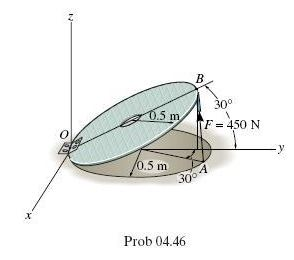×
Get Full Access to Engineering Mechanics: Statics - 14 Edition - Chapter 4 - Problem 4-50
Get Full Access to Engineering Mechanics: Statics - 14 Edition - Chapter 4 - Problem 4-50

×

# Answer: Strut AB of the 1-m-diameter hatch door exerts aISBN: 9780133918922 126

## Solution for problem 4-50 Chapter 4

Engineering Mechanics: Statics | 14th Edition

• Textbook Solutions
• 2901 Step-by-step solutions solved by professors and subject experts
• Get 24/7 help from StudySoup virtual teaching assistantsEngineering Mechanics: Statics | 14th Edition

4 5 1 306 Reviews
22
4
Problem 4-50

Strut AB of the 1-m-diameter hatch door exerts a force of 450 N on point B. Determine the moment of this force about point O. x z y F 450 N 0.5 m A B O 30 30 0.5 m Prob. 450

Step-by-Step Solution:
Step 1 of 3

Problem 4-50

Strut AB of the 1-m-diameter hatch door exerts a force of 450 N on point B. Determine the moment of this force about point O.Step-by-Step Solution

Step  1 of 3

First define vectors:Step 2 of 3

Step 3 of 3

##### ISBN: 9780133918922

The full step-by-step solution to problem: 4-50 from chapter: 4 was answered by , our top Engineering and Tech solution expert on 11/10/17, 05:25PM. This full solution covers the following key subjects: Force, point, hatch, exerts, determine. This expansive textbook survival guide covers 11 chapters, and 1136 solutions. This textbook survival guide was created for the textbook: Engineering Mechanics: Statics, edition: 14. Engineering Mechanics: Statics was written by and is associated to the ISBN: 9780133918922. Since the solution to 4-50 from 4 chapter was answered, more than 308 students have viewed the full step-by-step answer. The answer to “Strut AB of the 1-m-diameter hatch door exerts a force of 450 N on point B. Determine the moment of this force about point O. x z y F 450 N 0.5 m A B O 30 30 0.5 m Prob. 450” is broken down into a number of easy to follow steps, and 42 words.

Unlock Textbook Solution